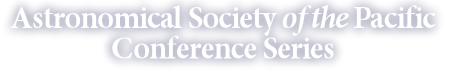Paper: Physical Quantities, Measurement Sets, Theories Volume: 461, Astronomical Data Analysis Software and Systems XXI Page: 145 Authors: Viallefond, F. Abstract: A methodology is proposed to develop efficient, robust and expressive data models. The idea is to transform objects described using our human language into mathematical objects which can then be used efficiently in information systems. This is done using topological spaces and algebras to model data types. Technically it is implemented using parametric polymorphism. Two examples are shown, 1) a simple well known object, the physical quantities, and 2) a data-base object, the measurement sets which bind the measurements to their experimental contexts. This leads to theories. The result is high expressiveness by formulating equations and data base operations by means of λ calculi. The theory of the measurement set encapsulates the relational model. Using topoi it is a generalization, a category above the sets.